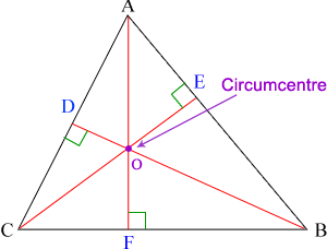# Perpendicular Bisectors, Angle Bisectors, and Circumcenters

## Follow these steps to construct perpendicular bisectors

1) Using the COMPASS TOOL, create a circle with radius AB and center point A 2) Using the COMPASS TOOL, create a circle with radius AB and center point B 3) Using the SEGMENT TOOL, draw a segment that connects the intersections of circles A and B 4) Using the POINT TOOL, mark point E at the intersection of segments AB and CD RESULTS: ﻿Segment CD is the Perpendicular Bisector of segment AB Point E is the Midpoint of segment AB

## Construction #1

What does the term perpendicular bisector mean?

Select all that apply
• A
• B
• C

## Follow these steps to bisect an angle:

1) Using the POINT TOOL, mark point D on segment AB 2) Using the COMPASS TOOL, create a circle with radius AD and center point A 3) Using the POINT TOOL, mark point F where circle A intersects segment AC 4) Using the COMPASS TOOL, create a circle with the radius DF and center point D 5) Using the COMPASS TOOL, create a circle with the radius DF and center point F 6) Using the SEGMENT TOOL, draw a segment from point A to the intersection of circles D and F RESULTS: Segment AG is the Angle Bisector of angle CAB

What does the term angle bisector mean?

Select all that apply
• A
• B
• C

## The Circumcenter of a TriangleThe center of a triangle's circumcircle. It is where the "perpendicular bisectors" (lines that are at right angles to the midpoint of each side) meet.The circumcenter of a triangle can be in different places based on the type of triangle.

The circumcenter is the intersection of which 3 lines in a triangle?

Select all that apply
• A
• B
• C
• D